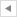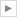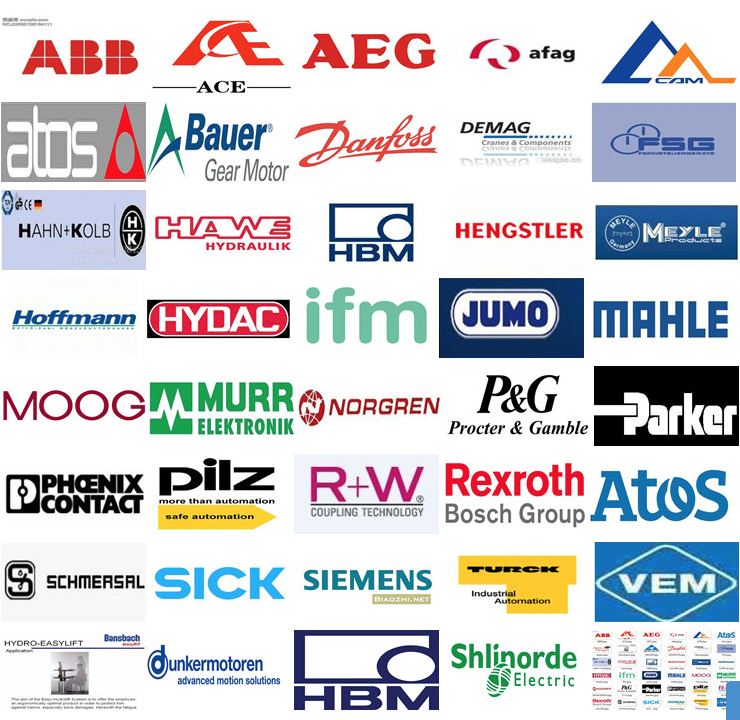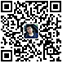• 产品分类
联系我们无锡远路贸易有限公司

咨询热线：0510-88710854
邮箱：yuanlu.company@163.com

关注排行
• P-T
您的位置：
• # 美国SQUARE-D美商实快

类别：P-T

• 型号：6464212

• 价格：￥1980.00

• 发布时间：2023-6-7 19:36:35

• 简介： 美国SQUARE-D美商实快

产品订购热线：18921392057
其他人还看了Other products
• 相关产品
• 产品描述
• 在线下单
• 在线留言

施耐德电气是全球更大的低压电器供应商,旗下有梅兰日兰、TE电器和美商实快电力三大品牌。 美商实快电力的历史：1902年：底特律熔断器制造厂成立，该厂主要生产箱式熔断器及盒式熔断器式开关。在公司导管接线盒上的署名《D》拥有很高的声望，因此，公司决定更名为实快电力（SquareD）；2002年，实快电力推出了世界上更小的Masterpact NT断路器和PowerBus225低敏感度布线系统；2003年美商实快电力品牌举办了公司成立100周年庆典。这100年同时也是电力和自动化管理技术发展与创新的100年；美商实快电力公司作为世界更优秀的电气产品，服务于美国各大公司及电力设施，并在世界各地遍布企业和销售网络，凭借近百年生产经验的配电设备品牌，它承诺创新和可靠的产品来迎合客户需要。在输配电及工控及自动化，广泛用于商业，建筑及工业各领域。美商实快电力的核心业务是电力与自动化管理。实快电力始终处在创新前沿，能够对全球的市场需求做出迅速反应，为配电和工业控制自动化领域提供符合NEMA标准的产品、系统与服务。该品牌产品具有5大特点：精巧、创新、优质、可靠、安全。SQUARE-D 989216
SQUARE-D 989270
SQUARE-D 989290
SQUARE-D 989315
SQUARE-D 989316
SQUARE-D 989330
SQUARE-D 989340
SQUARE-D 989350
SQUARE-D 989370
SQUARE-D 992115
SQUARE-D 992120
SQUARE-D 992125
SQUARE-D 992130
SQUARE-D 992140
SQUARE-D 992150
SQUARE-D 992215
SQUARE-D 992220
SQUARE-D 992225
SQUARE-D 992230
SQUARE-D 992235
SQUARE-D 992240
SQUARE-D 992250
SQUARE-D 992270
SQUARE-D 992315
SQUARE-D 992320
SQUARE-D 992325
SQUARE-D 992330
SQUARE-D 992335
SQUARE-D 992340
SQUARE-D 992350
SQUARE-D 992915
SQUARE-D 992920
SQUARE-D 997215
SQUARE-D 997216
SQUARE-D 997217
SQUARE-D 997218
SQUARE-D 997219
SQUARE-D 997226
SQUARE-D 997227
SQUARE-D 997316
SQUARE-D 997317
SQUARE-D 997318
SQUARE-D 997319
SQUARE-D 997326
SQUARE-D 997327
SQUARE-D 997370
SQUARE-D 999215
SQUARE-D 999216
SQUARE-D 999220
SQUARE-D 999230
SQUARE-D 999240
SQUARE-D 999250
SQUARE-D 999260
SQUARE-D 999270
SQUARE-D 999280
SQUARE-D 999290
SQUARE-D 999315
SQUARE-D 999316
SQUARE-D 999320
SQUARE-D 999330
SQUARE-D 999340
SQUARE-D 999350
SQUARE-D 999360
SQUARE-D 999370
SQUARE-D 999380
SQUARE-D 999390
SQUARE-D A1B1100
SQUARE-D A1B115
SQUARE-D A1B120
SQUARE-D A1B130
SQUARE-D A1B140
SQUARE-D A1B150
SQUARE-D A1B160
SQUARE-D A1B170
SQUARE-D A1B2100
SQUARE-D A1B215
SQUARE-D A1B220
SQUARE-D A1B230
SQUARE-D A1B240
SQUARE-D A1B250
SQUARE-D A1B260
SQUARE-D A1B270
SQUARE-D A1B280
SQUARE-D A1B290
SQUARE-D A1B3100
SQUARE-D A1B315
SQUARE-D A1B320
SQUARE-D A1B325
SQUARE-D A1B330
SQUARE-D A1B335
SQUARE-D A1B340
SQUARE-D A1B350
SQUARE-D A1B360
SQUARE-D A1B370
SQUARE-D A1B380
SQUARE-D A1B390
SQUARE-D A1L115
SQUARE-D A1L120
SQUARE-D A1L125
SQUARE-D A1L130
SQUARE-D A1L140
SQUARE-D A1L150
SQUARE-D A1L160
SQUARE-D A1L170
SQUARE-D A1L2100
SQUARE-D A1L215
SQUARE-D A1L220
SQUARE-D A1L225
SQUARE-D A1L230
SQUARE-D A1L240
SQUARE-D A1L250
SQUARE-D A1L260
SQUARE-D A1L270
SQUARE-D A1L280
SQUARE-D A1L290
SQUARE-D A1L3100
SQUARE-D A1L315
SQUARE-D A1L320
SQUARE-D A1L330
SQUARE-D A1L340
SQUARE-D A1L350
SQUARE-D A1L360
SQUARE-D A1L370
SQUARE-D A1L380
SQUARE-D A1L390
SQUARE-D B125
SQUARE-D EDB14015
SQUARE-D EDB14020
SQUARE-D EDB14025
SQUARE-D EDB14030
SQUARE-D EDB14035
SQUARE-D EDB14040
SQUARE-D EDB14045
SQUARE-D EDB14050
SQUARE-D EDB14060
SQUARE-D EDB14070
SQUARE-D EDB14100
SQUARE-D EDB24015
SQUARE-D EDB24020
SQUARE-D EDB24025
SQUARE-D EDB24030
SQUARE-D EDB24035
SQUARE-D EDB24040
SQUARE-D EDB24045
SQUARE-D EDB24050
SQUARE-D EDB24060
SQUARE-D EDB24070
SQUARE-D EDB24080
SQUARE-D EDB24090
SQUARE-D EDB24100
SQUARE-D EDB24110
SQUARE-D EDB24125
SQUARE-D EDB34015
SQUARE-D EDB34020
SQUARE-D EDB34025
SQUARE-D EDB34030
SQUARE-D EDB34035
SQUARE-D EDB34040
SQUARE-D EDB34050
SQUARE-D EDB34060
SQUARE-D EDB34070
SQUARE-D EDB34080
SQUARE-D EDB34090
SQUARE-D EDB34100
SQUARE-D EDB34110
SQUARE-D EDB34125
SQUARE-D EGB14015
SQUARE-D EGB14020
SQUARE-D EGB14025
SQUARE-D EGB14030
SQUARE-D EGB14035
SQUARE-D EGB14040
SQUARE-D EGB14045
SQUARE-D EGB14050
SQUARE-D EGB14060
SQUARE-D EGB14070
SQUARE-D EGB24015
SQUARE-D EGB24020
SQUARE-D EGB24025
SQUARE-D EGB24030
SQUARE-D EGB24035
SQUARE-D EGB24040
SQUARE-D EGB24045
SQUARE-D EGB24050
SQUARE-D EGB24060
SQUARE-D EGB24070
SQUARE-D EGB24080
SQUARE-D EGB24090
SQUARE-D EGB24100
SQUARE-D EGB24110
SQUARE-D EGB24125
SQUARE-D EGB34015
SQUARE-D EGB34020
SQUARE-D EGB34025
SQUARE-D EGB34030
SQUARE-D EGB34035
SQUARE-D EGB34040
SQUARE-D EGB34050
SQUARE-D EGB34060
SQUARE-D EGB34070
SQUARE-D EGB34080
SQUARE-D EGB34090
SQUARE-D EGB34100
SQUARE-D EGB34110
SQUARE-D EGB34125
SQUARE-D EH14015
SQUARE-D EH14020
SQUARE-D EH14025
SQUARE-D EH14030
SQUARE-D EH14040
SQUARE-D EH14045
SQUARE-D EH14050
SQUARE-D EH14060
SQUARE-D EH24015
SQUARE-D EH24020
SQUARE-D EH24025
SQUARE-D EH24030
SQUARE-D EH24035
SQUARE-D EH24040
SQUARE-D EH24045
SQUARE-D EH24050
SQUARE-D EH24060
SQUARE-D EH24070
SQUARE-D EH24080
SQUARE-D EH24090
SQUARE-D EH24100
SQUARE-D EH34015
SQUARE-D EH34020
SQUARE-D EH34025
SQUARE-D EH34030
SQUARE-D EH34035
SQUARE-D EH34040
SQUARE-D EH34050
SQUARE-D EH34060
SQUARE-D EH34070
SQUARE-D EH34080
SQUARE-D EH34090
SQUARE-D EH34100
SQUARE-D EHB14015
SQUARE-D EHB14020
SQUARE-D EHB14025
SQUARE-D EHB14030
SQUARE-D EHB14035
SQUARE-D EHB14040
SQUARE-D EHB14045
SQUARE-D EHB14050
SQUARE-D EHB14060
SQUARE-D EHB24015
SQUARE-D EHB24020
SQUARE-D EHB24025
SQUARE-D EHB24030
SQUARE-D EHB24035
SQUARE-D EHB24040
SQUARE-D EHB24045
SQUARE-D EHB24050
SQUARE-D EHB24060
SQUARE-D EHB24070
SQUARE-D EHB24080
SQUARE-D EHB24090
SQUARE-D EHB24100
SQUARE-D EHB34015
SQUARE-D EHB34020
SQUARE-D EHB34025
SQUARE-D EHB34030
SQUARE-D EHB34035
SQUARE-D EHB34040
SQUARE-D EHB34050
SQUARE-D EHB34060
SQUARE-D EHB34070
SQUARE-D EHB34080
SQUARE-D EHB34090
SQUARE-D EHB34100
SQUARE-D EJB14015
SQUARE-D EJB14020
SQUARE-D EJB14025
SQUARE-D EJB14030
SQUARE-D EJB14035
SQUARE-D EJB14040
SQUARE-D EJB14045
SQUARE-D EJB14050
SQUARE-D EJB14060
SQUARE-D EJB14070
SQUARE-D EJB24015
SQUARE-D EJB24020
SQUARE-D EJB24025
SQUARE-D EJB24030
SQUARE-D EJB24035
SQUARE-D EJB24040
SQUARE-D EJB24045
SQUARE-D EJB24050
SQUARE-D EJB24060
SQUARE-D EJB24070
SQUARE-D EJB34015
SQUARE-D EJB34020
SQUARE-D EJB34025
SQUARE-D EJB34030
SQUARE-D EJB34035
SQUARE-D EJB34040
SQUARE-D EJB34050
SQUARE-D EJB34060
SQUARE-D EJB34070
SQUARE-D EJB34080
SQUARE-D EJB34090
SQUARE-D EJB34100
SQUARE-D EJB34110
SQUARE-D EJB34125
SQUARE-D FA14015
SQUARE-D FA14015A
SQUARE-D FA14015B
SQUARE-D FA14015C
SQUARE-D FA14020
SQUARE-D FA14020A
SQUARE-D FA14020B
SQUARE-D FA14020C
SQUARE-D FA14030
SQUARE-D FA14030A
SQUARE-D FA14030B
SQUARE-D FA14030C
SQUARE-D FA14035A
SQUARE-D FA14035B
SQUARE-D FA14035C
SQUARE-D FA14040
SQUARE-D FA14040A
SQUARE-D FA14040B
SQUARE-D FA14040C
SQUARE-D FA14045A
SQUARE-D FA14045B
SQUARE-D FA14045C
SQUARE-D FA14050
SQUARE-D FA14050A
SQUARE-D FA14050B
SQUARE-D FA14050C
SQUARE-D FA14060
SQUARE-D FA14060A
SQUARE-D FA14060B
SQUARE-D FA14060C
SQUARE-D FA14070
SQUARE-D FA14070A
SQUARE-D FA14070B
SQUARE-D FA14070C
SQUARE-D FA14080A
SQUARE-D FA14090A
SQUARE-D FA14100
SQUARE-D FA14100A
SQUARE-D FA14100B
SQUARE-D FA14100C
SQUARE-D FA22015
SQUARE-D FA22015AB
SQUARE-D FA22015AC
SQUARE-D FA22015BC
SQUARE-D FA22020
SQUARE-D FA22020AB
SQUARE-D FA22020AC
SQUARE-D FA22020BC
SQUARE-D FA22025
SQUARE-D FA22025AB
SQUARE-D FA22025AC
SQUARE-D FA22030
SQUARE-D FA22030AB
SQUARE-D FA22030AC
SQUARE-D FA22030BC
SQUARE-D FA22035AB
SQUARE-D FA22035AC
SQUARE-D FA22040
SQUARE-D FA22040AB
SQUARE-D FA22040AC
SQUARE-D FA22040BC
SQUARE-D FA22045
SQUARE-D FA22045AC
SQUARE-D FA22045BC
SQUARE-D FA22050
SQUARE-D FA22050AB
SQUARE-D FA22050AC
SQUARE-D FA22050BC
SQUARE-D FA22060
SQUARE-D FA22060AB
SQUARE-D FA22060AC
SQUARE-D FA22060BC
SQUARE-D FA22070
SQUARE-D FA22070AB
SQUARE-D FA22070AC
SQUARE-D FA22070BC
SQUARE-D FA22080
SQUARE-D FA22090
SQUARE-D FA22090AB
SQUARE-D FA22090AC
SQUARE-D FA22090BC
SQUARE-D FA22100
SQUARE-D FA22100AB
SQUARE-D FA22100AC
SQUARE-D FA22100BC
SQUARE-D FA24015
SQUARE-D FA24015AB
SQUARE-D FA24015AC
SQUARE-D FA24015BC
SQUARE-D FA24020
SQUARE-D FA24020AB
SQUARE-D FA24020AC
SQUARE-D FA24020BC
SQUARE-D FA24025
SQUARE-D FA24025AB
SQUARE-D FA24025AC
SQUARE-D FA24025BC
SQUARE-D FA24030
SQUARE-D FA24030AB
SQUARE-D FA24030AC
SQUARE-D FA24030BC
SQUARE-D FA24035AB
SQUARE-D FA24035AC
SQUARE-D FA24040
SQUARE-D FA24040AB
SQUARE-D FA24040AC
SQUARE-D FA24040BC
SQUARE-D FA24045AB
SQUARE-D FA24045AC
SQUARE-D FA24050
SQUARE-D FA24050AB
SQUARE-D FA24050AC
SQUARE-D FA24050BC
SQUARE-D FA24060
SQUARE-D FA24060AB
SQUARE-D FA24060AC
SQUARE-D FA24060BC
SQUARE-D FA24070
SQUARE-D FA24070AB
SQUARE-D FA24070AC
SQUARE-D FA24070BC
SQUARE-D FA24080
SQUARE-D FA24080AB
SQUARE-D FA24080AC
SQUARE-D FA24080BC
SQUARE-D FA24090
SQUARE-D FA24090AB
SQUARE-D FA24090AC
SQUARE-D FA24090BC
SQUARE-D FA24100
SQUARE-D FA24100AB
SQUARE-D FA24100AC
SQUARE-D FA24100BC
SQUARE-D FA26015
SQUARE-D FA26015AB
SQUARE-D FA26015AC
SQUARE-D FA26015BC
SQUARE-D FA26020
SQUARE-D FA26020AB
SQUARE-D FA26020AC
SQUARE-D FA26020BC
SQUARE-D FA26025AB
SQUARE-D FA26025AC
SQUARE-D FA26025BC
SQUARE-D FA26030
SQUARE-D FA26030AB
SQUARE-D FA26030AC
SQUARE-D FA26030BC
SQUARE-D FA26035
SQUARE-D FA26035AC
SQUARE-D FA26035BC
SQUARE-D FA26040
SQUARE-D FA26040AB
SQUARE-D FA26040AC
SQUARE-D FA26040BC
SQUARE-D FA26050
SQUARE-D FA26050AB
SQUARE-D FA26050AC
SQUARE-D FA26050BC
SQUARE-D FA26060
SQUARE-D FA26060AB
SQUARE-D FA26060AC
SQUARE-D FA26060BC
SQUARE-D FA26070
SQUARE-D FA26070AB
SQUARE-D FA26070AC
SQUARE-D FA26070BC
SQUARE-D FA26080
SQUARE-D FA26080AB
SQUARE-D FA26080AC
SQUARE-D FA26080BC
SQUARE-D FA26090
SQUARE-D FA26090AB
SQUARE-D FA26090AC
SQUARE-D FA26090BC
SQUARE-D FA26100
SQUARE-D FA26100AB
SQUARE-D FA26100AC
SQUARE-D FA26100BC
SQUARE-D FA32015
SQUARE-D FA32020
SQUARE-D FA32025
SQUARE-D FA32030
SQUARE-D FA32035
SQUARE-D FA32040
SQUARE-D FA32050
SQUARE-D FA32060
SQUARE-D FA32070
SQUARE-D FA32080
SQUARE-D FA32090
SQUARE-D FA32100
SQUARE-D FA34015
SQUARE-D FA34020
SQUARE-D FA34025
SQUARE-D FA34030
SQUARE-D FA34035
SQUARE-D FA34040
SQUARE-D FA34050
SQUARE-D FA34060
SQUARE-D FA34070
SQUARE-D FA34080
SQUARE-D FA34090
SQUARE-D FA34100
SQUARE-D FA36007
SQUARE-D FA36015
SQUARE-D FA36020
SQUARE-D FA36025
SQUARE-D FA36030
SQUARE-D FA36035
SQUARE-D FA36040
SQUARE-D FA36050
SQUARE-D FA36060
SQUARE-D FA36070
SQUARE-D FA36080
SQUARE-D FA36090
SQUARE-D FA36100
SQUARE-D FAL12015
SQUARE-D FAL12020
SQUARE-D FAL12025
SQUARE-D FAL12030
SQUARE-D FAL12035
SQUARE-D FAL12040
SQUARE-D FAL12045
SQUARE-D FAL12050
SQUARE-D FAL12060
SQUARE-D FAL12070
SQUARE-D FAL12080
SQUARE-D FAL12090
SQUARE-D FAL12100
SQUARE-D FAL14015
SQUARE-D FAL14020
SQUARE-D FAL14025
SQUARE-D FAL14030
SQUARE-D FAL14035
SQUARE-D FAL14040
SQUARE-D FAL14045
SQUARE-D FAL14050
SQUARE-D FAL14060
SQUARE-D FAL14070
SQUARE-D FAL14080
SQUARE-D FAL14090
SQUARE-D FAL14100
SQUARE-D FAL22000
SQUARE-D FAL22015
SQUARE-D FAL22020
SQUARE-D FAL22025
SQUARE-D FAL22030
SQUARE-D FAL22035
SQUARE-D FAL22040
SQUARE-D FAL22040
SQUARE-D FAL22050
SQUARE-D FAL22060
SQUARE-D FAL22070
SQUARE-D FAL22080
SQUARE-D FAL22090
SQUARE-D FAL22100
SQUARE-D FAL24015
SQUARE-D FAL24020
SQUARE-D FAL24025
SQUARE-D FAL24030
SQUARE-D FAL24035
SQUARE-D FAL24040
SQUARE-D FAL24045
SQUARE-D FAL24050
SQUARE-D FAL24060
SQUARE-D FAL24070
SQUARE-D FAL24080
SQUARE-D FAL24090
SQUARE-D FAL24100
SQUARE-D FAL26003
SQUARE-D FAL26015
SQUARE-D FAL26020
SQUARE-D FAL26025
SQUARE-D FAL26030
SQUARE-D FAL26035
SQUARE-D FAL26040
SQUARE-D FAL26045
SQUARE-D FAL26050
SQUARE-D FAL26060
SQUARE-D FAL26070
SQUARE-D FAL26080
SQUARE-D FAL26090
SQUARE-D FAL26100
SQUARE-D FAL32015
SQUARE-D FAL32020
SQUARE-D FAL32025
SQUARE-D FAL32030
SQUARE-D FAL32035
SQUARE-D FAL32040
SQUARE-D FAL32050
SQUARE-D FAL32060
SQUARE-D FAL32070
SQUARE-D FAL32080
SQUARE-D FAL32090
SQUARE-D FAL32100
SQUARE-D FAL34000
SQUARE-D FAL34015
SQUARE-D FAL34020
SQUARE-D FAL34025
SQUARE-D FAL34030
SQUARE-D FAL34035
SQUARE-D FAL34040
SQUARE-D FAL34050
SQUARE-D FAL34060
SQUARE-D FAL34070
SQUARE-D FAL34080
SQUARE-D FAL34090
SQUARE-D FAL34090
SQUARE-D FAL34100
SQUARE-D FAL36000
SQUARE-D FAL3600311M
SQUARE-D FAL3600712M
SQUARE-D FAL36010
SQUARE-D FAL36015
SQUARE-D FAL3601513M
SQUARE-D FAL36020
SQUARE-D FAL36025
SQUARE-D FAL36030
SQUARE-D FAL3603013
SQUARE-D FAL3603015M
SQUARE-D FAL36035
SQUARE-D FAL36040
SQUARE-D FAL36050
SQUARE-D FAL3605014
SQUARE-D FAL3605014M
SQUARE-D FAL3605016
SQUARE-D FAL36060
SQUARE-D FAL36070
SQUARE-D FAL36080
SQUARE-D FAL36090
SQUARE-D FAL36100
SQUARE-D FAL3610016
SQUARE-D FAL3610018
SQUARE-D FAL3610018M
SQUARE-D FAL3615024M
SQUARE-D FAL3805016M
SQUARE-D FCL24015
SQUARE-D FCL24020
SQUARE-D FCL24025
SQUARE-D FCL24030
SQUARE-D FCL24035
SQUARE-D FCL24040
SQUARE-D FCL24050
SQUARE-D FCL24060
SQUARE-D FCL24070
SQUARE-D FCL24090
SQUARE-D FCL24100
SQUARE-D FCL34025
SQUARE-D FCL34035
SQUARE-D FGA34015
SQUARE-D FGA34020
SQUARE-D FGA34030
SQUARE-D FGA34040
SQUARE-D FGA34050
SQUARE-D FGA34060
SQUARE-D FGA34070
SQUARE-D FGA34080
SQUARE-D FGA34090
SQUARE-D FGA34100
SQUARE-D FH16015A
SQUARE-D FH16015B
SQUARE-D FH16015C
SQUARE-D FH16020A
SQUARE-D FH16020B
SQUARE-D FH16020C
SQUARE-D FH16025A
SQUARE-D FH16025B
SQUARE-D FH16025C
SQUARE-D FH16030A
SQUARE-D FH16030B
SQUARE-D FH16030C
SQUARE-D FH16035A
SQUARE-D FH16040A
SQUARE-D FH16040B
SQUARE-D FH16040C
SQUARE-D FH16050A
SQUARE-D FH16060A
SQUARE-D FH16060B
SQUARE-D FH16070A
SQUARE-D FH16100A
SQUARE-D FH16100C
SQUARE-D FH26015AC
SQUARE-D FH26015BC
SQUARE-D FH26015CA
SQUARE-D FH26020AC
SQUARE-D FH26020BC
SQUARE-D FH26020CA
SQUARE-D FH26025AC
SQUARE-D FH26030AC
SQUARE-D FH26030BC
SQUARE-D FH26030CA
SQUARE-D FH26035AC
SQUARE-D FH26035BC
SQUARE-D FH26040AC
SQUARE-D FH26040BC
SQUARE-D FH26040CA
SQUARE-D FH26050AC
SQUARE-D FH26050BC
SQUARE-D FH26050CA
SQUARE-D FH26060AC
SQUARE-D FH26060BC
SQUARE-D FH26060CA
SQUARE-D FH26070AC
SQUARE-D FH26070BC
SQUARE-D FH26080AC
SQUARE-D FH26080BC
SQUARE-D FH26090AC
SQUARE-D FH26090BC
SQUARE-D FH26100AC
SQUARE-D FH26100BC
SQUARE-D FH36015
SQUARE-D FH36020
SQUARE-D FH36025
SQUARE-D FH36030
SQUARE-D FH36035
SQUARE-D FH36040
SQUARE-D FH36050
SQUARE-D FH36060
SQUARE-D FH36070
SQUARE-D FH36080
SQUARE-D FH36090
SQUARE-D FH36100
SQUARE-D FHL16015
SQUARE-D FHL16020
SQUARE-D FHL16030
SQUARE-D FHL16040
SQUARE-D FHL16050
SQUARE-D FHL16060
SQUARE-D FHL16080
SQUARE-D FHL16100
SQUARE-D FHL26015
SQUARE-D FHL26020
SQUARE-D FHL26025
SQUARE-D FHL26030
SQUARE-D FHL26035
SQUARE-D FHL26040
SQUARE-D FHL26050
SQUARE-D FHL26060
SQUARE-D FHL26070
SQUARE-D FHL26080
SQUARE-D FHL26090
SQUARE-D FHL26100
SQUARE-D FHL36015
SQUARE-D FHL36020
SQUARE-D FHL36025
SQUARE-D FHL36030
SQUARE-D FHL36035
SQUARE-D FHL36040
SQUARE-D FHL36050
SQUARE-D FHL36060
SQUARE-D FHL36070
SQUARE-D FHL36080
SQUARE-D FHL36090
SQUARE-D FHL36100
SQUARE-D FHP16015
SQUARE-D FHP16020
SQUARE-D FHP16030
SQUARE-D FHP16040
SQUARE-D FHP16050
SQUARE-D FHP16060
SQUARE-D FHP16080
SQUARE-D FHP16100
SQUARE-D FHP26015
SQUARE-D FHP26020
SQUARE-D FHP26030
SQUARE-D FHP26040
SQUARE-D FHP26050
SQUARE-D FHP26060
SQUARE-D FHP26070
SQUARE-D FHP26090
SQUARE-D FHP26100
SQUARE-D FHP36015
SQUARE-D FHP36020
SQUARE-D FHP36025
SQUARE-D FHP36030
SQUARE-D FHP36035
SQUARE-D FHP36040
SQUARE-D FHP36050
SQUARE-D FHP3605016M
SQUARE-D FHP36060
SQUARE-D FHP36070
SQUARE-D FHP36080
SQUARE-D FHP36090
SQUARE-D FHP36100
SQUARE-D FI36020
SQUARE-D FI36030
SQUARE-D FI36040
SQUARE-D FI36050
SQUARE-D FI36060
SQUARE-D FI36070
SQUARE-D FI36080
SQUARE-D FI36090
SQUARE-D FI36100
SQUARE-D FJA34015
SQUARE-D FJA34020
SQUARE-D FJA34030
SQUARE-D FJA34040
SQUARE-D FJA34060
SQUARE-D FJA34070
SQUARE-D FJA34080
SQUARE-D FJA34090
SQUARE-D FJA34100
SQUARE-D FY12030A
SQUARE-D FY14015A
SQUARE-D FY14015B
SQUARE-D FY14015C
SQUARE-D FY14020A
SQUARE-D FY14020B
SQUARE-D FY14020C
SQUARE-D FY14025
SQUARE-D FY14025A
SQUARE-D FY14025B
SQUARE-D FY14025C
SQUARE-D FY14030A
SQUARE-D FY14030B
SQUARE-D FY14030C
SQUARE-D FY14040
SQUARE-D FY14050
SQUARE-D FY14060
SQUARE-D FY14070
SQUARE-D FY14090
SQUARE-D HOM115
SQUARE-D HOM115GFI
SQUARE-D HOM120
SQUARE-D HOM120GFI
SQUARE-D HOM125
SQUARE-D HOM130
SQUARE-D HOM140
SQUARE-D HOM150
SQUARE-D HOM2100
SQUARE-D HOM2125
SQUARE-D HOM215
SQUARE-D HOM220
SQUARE-D HOM225
SQUARE-D HOM230
SQUARE-D HOM235
SQUARE-D HOM240
SQUARE-D HOM245
SQUARE-D HOM250
SQUARE-D HOM260
SQUARE-D HOM270
SQUARE-D HOM280
SQUARE-D HOM290
SQUARE-D HOMT1515
SQUARE-D HOMT1515215
SQUARE-D HOMT1515220
SQUARE-D HOMT1515230
SQUARE-D HOMT1520
SQUARE-D HOMT2020
SQUARE-D HOMT2020220
SQUARE-D HOMT2020230
SQUARE-D KA26070AB
SQUARE-D KA26100
SQUARE-D KA26100AB
SQUARE-D KA26100AC
SQUARE-D KA26100BC
SQUARE-D KA26110AC
SQUARE-D KA26125
SQUARE-D KA26125AB
SQUARE-D KA26125AC
SQUARE-D KA26125BC
SQUARE-D KA26150
SQUARE-D KA26150AB
SQUARE-D KA26150AC
SQUARE-D KA26150BC
SQUARE-D KA26175
SQUARE-D KA26175AB
SQUARE-D KA26175AC
SQUARE-D KA26175BC
SQUARE-D KA26200
SQUARE-D KA26200AB
SQUARE-D KA26200AC
SQUARE-D KA26200BC
SQUARE-D KA26225
SQUARE-D KA26225AB
SQUARE-D KA26225AC
SQUARE-D KA26225BC
SQUARE-D KA26250
SQUARE-D KA26250AB
SQUARE-D KA26250AC
SQUARE-D KA26250BC
SQUARE-D KA36000
SQUARE-D KA36070
SQUARE-D KA36080
SQUARE-D KA36090
SQUARE-D KA36100
SQUARE-D KA36110
SQUARE-D KA36125
SQUARE-D KA36150
SQUARE-D KA36175
SQUARE-D KA36200
SQUARE-D KA36225
SQUARE-D KA36250
SQUARE-D KAL26070
SQUARE-D KAL26090
SQUARE-D KAL26100
SQUARE-D KAL26110
SQUARE-D KAL26125
SQUARE-D KAL26150
SQUARE-D KAL26175
SQUARE-D KAL26200
SQUARE-D KAL26225
SQUARE-D KAL26250
SQUARE-D KAL36000
SQUARE-D KAL36070
SQUARE-D KAL36080
SQUARE-D KAL36090
SQUARE-D KAL36100
SQUARE-D KAL36110
SQUARE-D KAL36125
SQUARE-D KAL36150
SQUARE-D KAL36175
SQUARE-D KAL36200
SQUARE-D KAL36225
SQUARE-D KAL36250
SQUARE-D KAL3625022M
SQUARE-D KAL3625025M
SQUARE-D KAL3625026M
SQUARE-D KAL3625029M
SQUARE-D KAL3625030M
SQUARE-D KAL3625031M
SQUARE-D KAL3625032M
SQUARE-D KAP26125
SQUARE-D KAP26150
SQUARE-D KAP26200
SQUARE-D KAP26225
SQUARE-D KAP36100
SQUARE-D KAP36110
SQUARE-D KAP36125
SQUARE-D KAP36150
SQUARE-D KAP36175
SQUARE-D KAP36200
SQUARE-D KAP36225
SQUARE-D KAP36250
SQUARE-D KC24125AB
SQUARE-D KC24125AC
SQUARE-D KC24125BC
SQUARE-D KC24150AB
SQUARE-D KC24150AC
SQUARE-D KC24150BC
SQUARE-D KC24175AB
SQUARE-D KC24175AC
SQUARE-D KC24175BC
SQUARE-D KC24200AB
SQUARE-D KC24200AC
SQUARE-D KC24200BC
SQUARE-D KC24250AB
SQUARE-D KC24250AC
SQUARE-D KC24250BC
SQUARE-D KC34110
SQUARE-D KC34125
SQUARE-D KC34150
SQUARE-D KC34175
SQUARE-D KC34200
SQUARE-D KC34225
SQUARE-D KC34250
SQUARE-D KCL24150
SQUARE-D KCL24225
SQUARE-D KCL34110
SQUARE-D KCL34125
SQUARE-D KCL34150
SQUARE-D KCL34175
SQUARE-D KCL34200
SQUARE-D KCL34225
SQUARE-D KCL34250
SQUARE-D KCP34110
SQUARE-D KCP34125
SQUARE-D KCP34150
SQUARE-D KCP34175
SQUARE-D KCP34200
SQUARE-D KCP34225
SQUARE-D KCP34250
SQUARE-D KDL32250
SQUARE-D KH26125AB
SQUARE-D KH26125AC
SQUARE-D KH26125BC
SQUARE-D KH26150AB
SQUARE-D KH26150AC
SQUARE-D KH26150BC
SQUARE-D KH26175AB
SQUARE-D KH26175AC
SQUARE-D KH26175BC
SQUARE-D KH26200AB
SQUARE-D KH26200AC
SQUARE-D KH26200BC
SQUARE-D KH26225AB
SQUARE-D KH26225AC
SQUARE-D KH26225BC
SQUARE-D KH26250AB
SQUARE-D KH26250AC
SQUARE-D KH26250BC
SQUARE-D KH36070
SQUARE-D KH36080
SQUARE-D KH36090
SQUARE-D KH36100
SQUARE-D KH36110
SQUARE-D KH36125
SQUARE-D KH36150
SQUARE-D KH36175
SQUARE-D KH36200
SQUARE-D KH36225
SQUARE-D KH36250
SQUARE-D KHL26070
SQUARE-D KHL26100
SQUARE-D KHL26125
SQUARE-D KHL26150
SQUARE-D KHL26175
SQUARE-D KHL26200
SQUARE-D KHL26225
SQUARE-D KHL26250
SQUARE-D KHL36000M
SQUARE-D KHL36000M1127
SQUARE-D KHL36070
SQUARE-D KHL36100
SQUARE-D KHL36110
SQUARE-D KHL36125
SQUARE-D KHL36150
SQUARE-D KHL36175
SQUARE-D KHL36200
SQUARE-D KHL36225
SQUARE-D KHL36250
SQUARE-D KHP36110
SQUARE-D KHP36125
SQUARE-D KHP36150
SQUARE-D KHP36175
SQUARE-D KHP36200
SQUARE-D KHP36225
SQUARE-D KHP36250
SQUARE-D KI26110AB
SQUARE-D KI26110AC
SQUARE-D KI26125AB
SQUARE-D KI26125BC
SQUARE-D KI26150AC
SQUARE-D KI26175AC
SQUARE-D KI26200
SQUARE-D KI26200AC
SQUARE-D KI26225AC
SQUARE-D KI36110
SQUARE-D KI361101021
SQUARE-D KI36125
SQUARE-D KI36150
SQUARE-D KI36175
SQUARE-D KI36200
SQUARE-D KI362001021
SQUARE-D KI36225
SQUARE-D KI362251021
SQUARE-D KI36225G
SQUARE-D KI36250
SQUARE-D KIL26110
SQUARE-D KIL26125
SQUARE-D KIL26150
SQUARE-D KIL26175
SQUARE-D KIL26200
SQUARE-D KIL26225
SQUARE-D KIL26250
SQUARE-D KIL36110
SQUARE-D KIL36125
SQUARE-D KIL36150
SQUARE-D KIL36175
SQUARE-D KIL36200
SQUARE-D KIL36225
SQUARE-D KIL36250
SQUARE-D KIP36125
SQUARE-D KIP36150
SQUARE-D KIP36175
SQUARE-D KIP36200
SQUARE-D KIP36225
SQUARE-D KIP36250
SQUARE-D LA26250
SQUARE-D LA26250AC
SQUARE-D LA26300
SQUARE-D LA26300AB
SQUARE-D LA26300AC
SQUARE-D LA26300BC
SQUARE-D LA26350
SQUARE-D LA26350AB
SQUARE-D LA26350AC
SQUARE-D LA26350BC
SQUARE-D LA26400
SQUARE-D LA26400AB
SQUARE-D LA26400AC
SQUARE-D LA26400BC
SQUARE-D LA36125
SQUARE-D LA36150
SQUARE-D LA36175
SQUARE-D LA36200
SQUARE-D LA36225
SQUARE-D LA36250
SQUARE-D LA36275
SQUARE-D LA36300
SQUARE-D LA36350
SQUARE-D LA36400
SQUARE-D LAL26125
SQUARE-D LAL26150
SQUARE-D LAL26175
SQUARE-D LAL26200
SQUARE-D LAL26225
SQUARE-D LAL26250
SQUARE-D LAL26300
SQUARE-D LAL26350
SQUARE-D LAL26400
SQUARE-D LAL36000
SQUARE-D LAL36000M
SQUARE-D LAL36125
SQUARE-D LAL36150
SQUARE-D LAL36175
SQUARE-D LAL36200
SQUARE-D LAL36225
SQUARE-D LAL36250
SQUARE-D LAL36300
SQUARE-D LAL36350
SQUARE-D LAL36400
SQUARE-D LAL3640022M
SQUARE-D LAL3640028M
SQUARE-D LAL3640030M
SQUARE-D LAL3640031M
SQUARE-D LAL3640032M
SQUARE-D LAL3640033M
SQUARE-D LAL3640035M
SQUARE-D LAL3640036M
SQUARE-D LAP26400
SQUARE-D LAP36200
SQUARE-D LAP36225
SQUARE-D LAP36250
SQUARE-D LAP36300
SQUARE-D LAP36350
SQUARE-D LAP36400
SQUARE-D LC26300AB
SQUARE-D LC26300AC
SQUARE-D LC26300BC
SQUARE-D LC26350AB
SQUARE-D LC26350AC
SQUARE-D LC26350BC
SQUARE-D LC26400AB
SQUARE-D LC26400AC
SQUARE-D LC26400BC
SQUARE-D LC26500AB
SQUARE-D LC26500AC
SQUARE-D LC26500BC
SQUARE-D LC26600AB
SQUARE-D LC26600AC
SQUARE-D LC26600BC
SQUARE-D LC36300
SQUARE-D LC36350
SQUARE-D LC36400
SQUARE-D LC36450
SQUARE-D LC36500
SQUARE-D LC36600
SQUARE-D LCL26400
SQUARE-D LCL26600
SQUARE-D LCL36300
SQUARE-D LCL36350
SQUARE-D LCL36400
SQUARE-D LCL36450
SQUARE-D LCL36500
SQUARE-D LCL36600
SQUARE-D LCP36350
SQUARE-D LCP36400
SQUARE-D LCP36500
SQUARE-D LCP36600
SQUARE-D LE36100LI
SQUARE-D LE36100LS
SQUARE-D LE36100LSG
SQUARE-D LE36150LSG
SQUARE-D LE36200LI
SQUARE-D LE36225LI
SQUARE-D LE36225LS
SQUARE-D LE36225LSG
SQUARE-D LE36250LI
SQUARE-D LE36250LSG
SQUARE-D LE36350LI
SQUARE-D LE36400LI
SQUARE-D LE36400LIG
SQUARE-D LE36400LS
SQUARE-D LE36400LSG
SQUARE-D LE36600LI
SQUARE-D LE36600LS
SQUARE-D LE36600LSG
SQUARE-D LEL36150LSG
SQUARE-D LEL36225LSG
SQUARE-D LEL36250LI
SQUARE-D LEL36300LI
SQUARE-D LEL36400LI
SQUARE-D LH26300AB
SQUARE-D LH26300AC
SQUARE-D LH26300BC
SQUARE-D LH26350AB
SQUARE-D LH26350AC
SQUARE-D LH26350BC
SQUARE-D LH26400AB
SQUARE-D LH26400AC
SQUARE-D LH26400BC
SQUARE-D LH36175
SQUARE-D LH36225
SQUARE-D LH36250
SQUARE-D LH36300
SQUARE-D LH36350
SQUARE-D LH36400
SQUARE-D LHL26200
SQUARE-D LHL26250
SQUARE-D LHL26300
SQUARE-D LHL26350
SQUARE-D LHL26400
SQUARE-D LHL36150
SQUARE-D LHL36175
SQUARE-D LHL36200
SQUARE-D LHL36225
SQUARE-D LHL36250
SQUARE-D LHL36300
SQUARE-D LHL36350
SQUARE-D LHL36400
SQUARE-D LHP26400
SQUARE-D LHP36300
SQUARE-D LHP36350
SQUARE-D LHP36400
SQUARE-D LI36300
SQUARE-D LI36350
SQUARE-D LI36400
SQUARE-D LI36450
SQUARE-D LI36500
SQUARE-D LI36600
SQUARE-D LIL36300
SQUARE-D LIL36350
SQUARE-D LIL36400
SQUARE-D LIL36450
SQUARE-D LIL36500
SQUARE-D LIL36600
SQUARE-D LX36100
SQUARE-D LX36100G
SQUARE-D LX36125
SQUARE-D LX36150
SQUARE-D LX36150G
SQUARE-D LX36175G
SQUARE-D LX36200
SQUARE-D LX36200G
SQUARE-D LX36225
SQUARE-D LX36250
SQUARE-D LX36250G
SQUARE-D LX36300
SQUARE-D LX36300G
SQUARE-D LX36400
SQUARE-D LX36400G
SQUARE-D LX36500
SQUARE-D LX36600
SQUARE-D LX36600G
SQUARE-D LXL36200
SQUARE-D LXL36200G
SQUARE-D LXL36400
SQUARE-D LXL36400G
SQUARE-D LXL36600G
SQUARE-D LXP36400
SQUARE-D LXP36400G
SQUARE-D LXP36600G
SQUARE-D MA26500AB
SQUARE-D MA26500AC
SQUARE-D MA26500BC
SQUARE-D MA26600AB
SQUARE-D MA26600AC
SQUARE-D MA26600BC
SQUARE-D MA26700AB
SQUARE-D MA26700AC
SQUARE-D MA26700BC
SQUARE-D MA26800AB
SQUARE-D MA26800AC
SQUARE-D MA26800BC
SQUARE-D MA36300
SQUARE-D MA36350
SQUARE-D MA36400
SQUARE-D MA36450
SQUARE-D MA36500
SQUARE-D MA36600
SQUARE-D MA36700
SQUARE-D MA36800
SQUARE-D MAL261000
SQUARE-D MAL26400
SQUARE-D MAL26450
SQUARE-D MAL26500
SQUARE-D MAL26600
SQUARE-D MAL26700
SQUARE-D MAL26800
SQUARE-D MAL26900
SQUARE-D MAL361000
SQUARE-D MAL361200
SQUARE-D MAL36200
SQUARE-D MAL36250
SQUARE-D MAL36300
SQUARE-D MAL36350
SQUARE-D MAL36400
SQUARE-D MAL36450
SQUARE-D MAL36500
SQUARE-D MAL36600
SQUARE-D MAL36700
SQUARE-D MAL36800
SQUARE-D MAL36900
SQUARE-D MAP26600
SQUARE-D MAP26800
SQUARE-D MAP36400
SQUARE-D MAP36450
SQUARE-D MAP36500
SQUARE-D MAP36600
SQUARE-D MAP36700
SQUARE-D MAP36800
SQUARE-D MAP36900
SQUARE-D ME36225LI
SQUARE-D ME36225LSG
SQUARE-D ME36300LSG
SQUARE-D ME36400LI
SQUARE-D ME36400LIG
SQUARE-D ME36400LS
SQUARE-D ME36400LSG
SQUARE-D ME36600LI
SQUARE-D ME36600LIG
SQUARE-D ME36600LS
SQUARE-D ME36600LSG
SQUARE-D ME36700LS
SQUARE-D ME36700LSG
SQUARE-D ME36800LI
SQUARE-D ME36800LI1021
SQUARE-D ME36800LIG
SQUARE-D ME36800LIG1021
SQUARE-D ME36800LS
SQUARE-D ME36800LSG
SQUARE-D MEL36400LSG
SQUARE-D MEL36500LS
SQUARE-D MEL36600LI
SQUARE-D MEL36600LSG
SQUARE-D MEL36800LI
SQUARE-D MEL36800LI1027
SQUARE-D MEL36800LS
SQUARE-D MEL36800LSG
SQUARE-D MEP36800LIG
SQUARE-D MEP36800LS
SQUARE-D MEP36800LSG
SQUARE-D MH36300
SQUARE-D MH36350
SQUARE-D MH36400
SQUARE-D MH36450
SQUARE-D MH36500
SQUARE-D MH36600
SQUARE-D MH36700
SQUARE-D MH36800
SQUARE-D MHL261000
SQUARE-D MHL26500
SQUARE-D MHL26600
SQUARE-D MHL26700
SQUARE-D MHL26800
SQUARE-D MHL26900
SQUARE-D MHL361000
SQUARE-D MHL36300
SQUARE-D MHL36350
SQUARE-D MHL36400
SQUARE-D MHL36450
SQUARE-D MHL36500
SQUARE-D MHL36600
SQUARE-D MHL36700
SQUARE-D MHL36800
SQUARE-D MHL36900
SQUARE-D MHP36400
SQUARE-D MHP36600
SQUARE-D MHP36800
SQUARE-D MX36250
SQUARE-D MX36250G
SQUARE-D MX36400
SQUARE-D MX36400G
SQUARE-D MX36600
SQUARE-D MX36600G
SQUARE-D MX36800
SQUARE-D MX36800G
SQUARE-D MXL36250
SQUARE-D MXL36450
SQUARE-D MXL36600
SQUARE-D MXL36600G
SQUARE-D MXL36800
SQUARE-D MXL36800G
SQUARE-D MXP36800G
SQUARE-D AL800SN
SQUARE-D NA361000
SQUARE-D NA361200
SQUARE-D NA36600
SQUARE-D NA36700
SQUARE-D NA36800
SQUARE-D NA36900
SQUARE-D NAL261000
SQUARE-D NAL261200
SQUARE-D NAL26600
SQUARE-D NAL26700
SQUARE-D NAL26800
SQUARE-D NAL26900
SQUARE-D NAL361000
SQUARE-D NAL361200
SQUARE-D NAL36600
SQUARE-D NAL36700
SQUARE-D NAL36800
SQUARE-D NAL36900
SQUARE-D NAP361200
SQUARE-D NC361000
SQUARE-D NC361200
SQUARE-D NC36600
SQUARE-D NC36700
SQUARE-D NC36800
SQUARE-D NC36900
SQUARE-D NCL261000
SQUARE-D NCL261200
SQUARE-D NCL26600
SQUARE-D NCL26700
SQUARE-D NCL26800
SQUARE-D NCL26900
SQUARE-D NCL361000
SQUARE-D NCL361200
SQUARE-D NCL36600
SQUARE-D NCL36700
SQUARE-D NCL36800
SQUARE-D NCL36900
SQUARE-D NE361000LS
SQUARE-D NE361000LSG
SQUARE-D NE361200LI
SQUARE-D NE361200LIG
SQUARE-D NE361200LS
SQUARE-D NE361200LSG
SQUARE-D NE36600LS
SQUARE-D NE36800LS
SQUARE-D NEL361000LI
SQUARE-D NEL361000LSG
SQUARE-D NEL361200LI
SQUARE-D NEL361200LS
SQUARE-D NEL361200LSG
SQUARE-D NHL261000
SQUARE-D NHL261200
SQUARE-D NHL361000
SQUARE-D NHL361200
SQUARE-D NHL3612001021
SQUARE-D NX361000G
SQUARE-D NX361200
SQUARE-D NX361200G
SQUARE-D NX361200G1021
SQUARE-D NX36800
SQUARE-D NX36800G
SQUARE-D NXL361000
SQUARE-D NXL361000G
SQUARE-D NXL361200
SQUARE-D NXL361200G
SQUARE-D NXL361200G1021
SQUARE-D NXP361000G
SQUARE-D NXP361200
SQUARE-D NXP361200G
SQUARE-D NXP36800
SQUARE-D PAF2026
SQUARE-D PAF2036
SQUARE-D PAF20361021
SQUARE-D PAF20361127
SQUARE-D PAF2036G
SQUARE-D PAL361000
SQUARE-D PAL361200
SQUARE-D PAL361600
SQUARE-D PAL361800
SQUARE-D PAL362000
SQUARE-D PAL36800
SQUARE-D PCF2536
SQUARE-D PCF2536G
SQUARE-D PEF361200LI
SQUARE-D PEF361200LSG
SQUARE-D PEF361600LI
SQUARE-D PEF361600LIG
SQUARE-D PEF361600LS
SQUARE-D PEF361600LSG
SQUARE-D PEF362000LI
SQUARE-D PEF362000LIG
SQUARE-D PEF362000LS
SQUARE-D PEF362000LSG
SQUARE-D PHF2036
SQUARE-D PHF2036G
SQUARE-D PXF361000G
SQUARE-D PXF361200
SQUARE-D PXF361200G
SQUARE-D PXF361400G
SQUARE-D PXF361600
SQUARE-D PXF361600G
SQUARE-D PXF362000
SQUARE-D PXF362000G
SQUARE-D PXF362500G
SQUARE-D Q12100
SQUARE-D Q12100TF
SQUARE-D Q12100VH
SQUARE-D Q12125
SQUARE-D Q1215
SQUARE-D Q12150
SQUARE-D Q1220
SQUARE-D
•• 18921392057

24小时客服热线